Updating search results...

# 5 Results

View
Selected filters:
• UT.MATH.III.A.SSE.1.b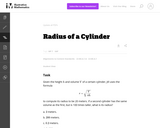Unrestricted Use
CC BY
Rating
0.0 stars

This is a task from the Illustrative Mathematics website that is one part of a complete illustration of the standard to which it is aligned. Each task has at least one solution and some commentary that addresses important asects of the task and its potential use. Here are the first few lines of the commentary for this task: Given the height $h$ and volume $V$ of a certain cylinder, Jill uses the formula r=\sqrt{\frac{V}{\pi h}} to compute its radius to be 20 meters. If a s...

Subject:
Mathematics
Secondary Mathematics
Material Type:
Activity/Lab
Provider:
Illustrative Mathematics
Provider Set:
Illustrative Mathematics
Author:
Illustrative Mathematics
03/20/2013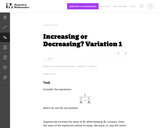Unrestricted Use
CC BY
Rating
0.0 stars

Students are asked to consider the expression that arises in physics as the combined resistance of two resistors in parallel. However, the context is not explicitly considered here. The task is good general preparation for problems more specifically aligned to either A-SSE.1 or A-SSE.2.

Subject:
Mathematics
Secondary Mathematics
Material Type:
Activity/Lab
Provider:
Illustrative Mathematics
Provider Set:
Illustrative Mathematics
Author:
Illustrative Mathematics
05/01/2012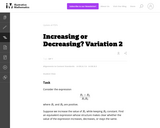Unrestricted Use
CC BY
Rating
0.0 stars

The purpose of this task is to help students see manipulation of expressions as an activity undertaken for a purpose.

Subject:
Mathematics
Secondary Mathematics
Material Type:
Activity/Lab
Provider:
Illustrative Mathematics
Provider Set:
Illustrative Mathematics
Author:
Illustrative Mathematics
05/01/2012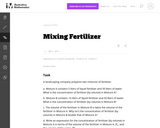Unrestricted Use
CC BY
Rating
0.0 stars

The problem deals with a rational expression which is built up from operations arising naturally in a context: adding the volumes of the fertilizer and the water, and dividing the volume of the fertilizer by the resulting sum. Thus it encourages students to see the expression as having meaning in terms of numbers and operations, rather than as an abstract arrangement of symbols.

Subject:
Mathematics
Secondary Mathematics
Material Type:
Activity/Lab
Provider:
Illustrative Mathematics
Provider Set:
Illustrative Mathematics
Author:
Illustrative Mathematics
05/01/2012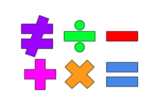Unrestricted Use
Public Domain
Rating
0.0 stars

This lesson is for a math classroom, but can be adapted to fit any grade, subject, or content.&nbsp;&nbsp;In this lesson, students will use an iPad and its features: Keynote, Pages, Garageband, Numbers, Presentation, and iMovie.&nbsp; Students will use 3 of those features/programs to create a video lesson consisting of several examples from a topic of their choice.&nbsp; This project is in place of a term final, so their chosen topic should be from their current term.Image citation: The image is one I created.&nbsp;&nbsp;

Subject:
Secondary Mathematics
Material Type:
Assessment
Author:
Tyrani Bevell